Next: Gravitational Waves from Coalescing Up: A Gravitational Wave Map Previous: A Gravitational Wave Map

### Gravitational waves from supernova explosions

Ā

The observed supernova frequencies of different types (SN Ia, Ib, II) in different types of galaxies (E-...-S-...-Ir) (see van den Bergh and Tammann, 1991) immediately provide us with the SN frequency distribution in space. We can investigate projection of this distribution onto the celestial sphere in two ways: in terms of lines of constant SN frequencies per one square degree for all galaxies from the sample (Figure 36), and in terms of constant GW amplitudes at a given SN frequency per square degree (Figure 44).

Ā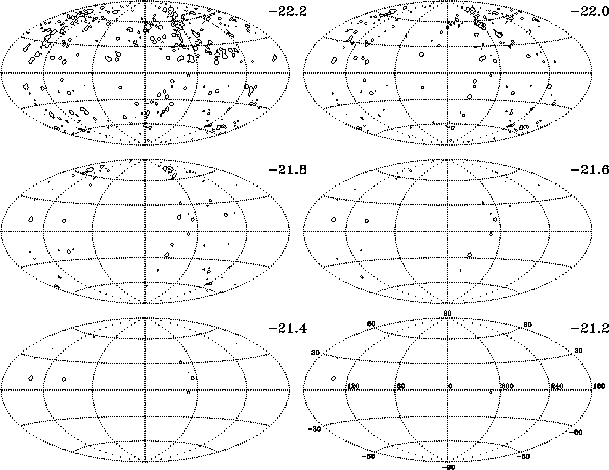Figure 44: Distribution of GW amplitudes from SN events over the sky. Inside regions encircled by the lines the total SN event rate >1/30Āyrdeg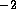at a given level of(Lipunov et al., 1995a).Ā

Inside regions encircled by the lines of constant SN frequency in FigureĀ45, the total SN frequency exceeds 1 per 3, 10, 30 and 100 year per square degree, respectively. This figure represents the cross-sections of the projected mass density per square degree by mass.

Ā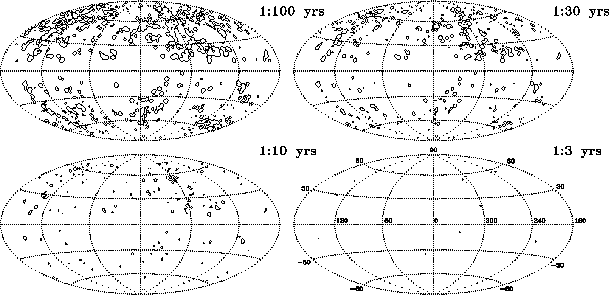Figure 45: The sky distribution of the total supernova frequency from nearby galaxies in terms of lines of equal frequencies per square degree. The total SN frequency exceeds 1 per 3, 10, 30 and 100 year per square degree inside regions encircled by the lines (Lipunov et al., 1995a). Ā

Inside regions encircled by the lines of constant(FigureĀ44), the total SN event rate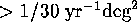at a given level of. In other words, the cross-sections of nearby galaxy space distribution by distance are given by this figure.

We assume after Thorne (1988) the characteristic strain metric amplitudeto be: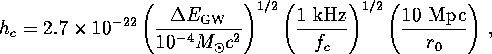where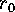is a distance to the source,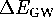is the total energy radiated as GW; a conservative estimate of GW energy during SN explosion0.1 percent of the expected neutrinoĀ luminosity is used. The characteristic frequency of the GW burstĀ is assumed to be 1 kHz. We must note that GW bursts produced by supernova explosions have been poorly understood so far, so only relative figures ofmay have meaning.

In FigureĀ46 the SN rates of different types integrated over the whole sky are plotted as a function of a given detector sensitivity in terms of the strain metric amplitude h. Clearly seen are contributions from the closest groups of galaxies and the Virgo cluster (assuming a Hubble constant of 75 km s). At better sensitivities () a power law with a slope of -3 is seen,, which reflects the almost isotropical distribution of matter beyond7ĀMpc. The total rate of SN events over the sky for nearby galaxies is about 40 per year.

ĀFigure 46: SNe rates of different types integrated over the whole sky as a function of a given detector sensitivity in terms of strain metric amplitude h. Contributions from some close groups of galaxies are indicated (Lipunov et al., 1995a).ĀNext: Gravitational Waves from Coalescing Up: A Gravitational Wave Map Previous: A Gravitational Wave Map

Mike E. Prokhorov
Sat Feb 22 18:38:13 MSK 1997